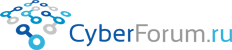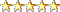Наши страницы
8Observer8
 Войти Регистрация Восстановить пароль
 Тесты Блоги Социальные группы Поиск Сообщения за день Все разделы прочитаны# Specs for Node.js Calculator using Jasmine and JavaScript 5

Запись от 8Observer8 размещена 24.02.2019 в 20:37

Let's write an executable documentation for server side calculator.

Specifications:
• "Add(a, b)" method must to sum positive numbers. Specification name: Add_SumPositiveNumbers_ReturnsSum
• "Sub(a, b)" method must to subtract positive numbers. Specification name: Sub_SubtractPositiveNumbers_ReturnsSub

Instruction:
• Create the "calculator-nodejs-jasmine-es5" folder
• Run these commands to install Jasmine locally:
Цитата:
npm init -y
npm install --save-dev jasmine
• Create the "jasmine.json" file in the "calculator-nodejs-jasmine-es5" folder
• Copy the content for the "jasmine.json" file from the link: https://jasmine.github.io/setup/nodejs.html and make some changes, see the content below:

jasmine.json

JSON
 ```1 2 3 4 5 6 7 8 ``` ```{     "spec_dir": "src_specs",     "spec_files": [         "**/*_tests.js",         "!**/*nospec.js"     ],     "random": false }```
• Open the "package.json" file and add the command to run tests:
JSON
 ```1 2 3 ``` ```    "scripts": {         "test": "node node_modules/jasmine/bin/jasmine.js  --config=jasmine.json"     },```
• Try to run tests. Enter the command:
Цитата:
npm run test
You will see a message that "No specs found"
• Create the "src_shared" folder. Create the "calculator.js" file the the "src_shared" folder
• Create the "src_specs" folder. Create the "calculator_tests.js" file the the "src_specs" folder
• Add our specs described above to the "calculator_tests.js" file:

calculator_tests.js
Javascript
 ```1 2 3 4 5 6 7 8 9 10 11 12 13 14 15 16 17 18 19 20 21 22 23 24 25 26 27 28 29 30 31 32 33 34 ``` ```var Calculator = require("../src_shared/calculator");   describe("Calculator", function() {     it("Add_SumPositiveNumbers_ReturnsSum", function()     {         // Arrange         var calculator = new Calculator();         var a = 5;         var b = 2;         var expectedSum = 7;           // Act         var actualSum = calculator.Add(a, b);           // Assert         expect(actualSum).toEqual(expectedSum);     });       it("Sub_SubtractPositiveNumbers_ReturnsSub", function()     {         // Arrange         var calculator = new Calculator();         var a = 5;         var b = 2;         var expectedSub = 3;           // Act         var actualSub = calculator.Sub(a, b);           // Assert         expect(actualSub).toEqual(expectedSub);     }); });```
• If you will run tests using the command "npm run test" then you will see the message:
Цитата:
ReferenceError: Calculator is not defined
• Let's implement these methods and run tests using the command "npm run test". You will see that the tests are passed

calculator.js

Javascript
 ```1 2 3 4 5 6 7 8 9 10 11 12 13 14 15 16 ``` ```var Calculator = function() {   };   Calculator.prototype.Add = function(a, b) {     return a + b; };   Calculator.prototype.Sub = function(a, b) {     return a - b; };   module.exports = Calculator;```Размещено в Без категории
Просмотров 99 Комментарии 0
Всего комментариев 0
Комментарии
 КиберФорум - форум программистов, компьютерный форум, программирование Реклама - Обратная связь
 Powered by vBulletin® Version 3.8.9Copyright ©2000 - 2019, vBulletin Solutions, Inc.